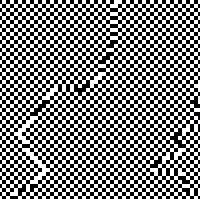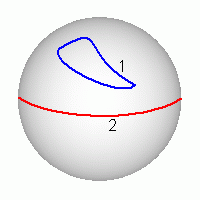# AntiferromagneticsIf the exchange interaction constant J < 0 , then for low temperature nearest spins are anti-aligned. In the simplest Ising antiferromagnetic on square grid they form two ordered sub-lattices (disposed as cells on a chess-board). Starting at random configuration you can see clusters of ordered phase formation and movement of domain walls. The Metropolis algorithm is used in the applet bellow.

Controls Click mouse to get a new spins configuration. Press "Enter" to set a new T value. You see the total magnetization M (the red curve) and energy E (the blue curve) in the right part of the applet and in the Status bar.

It is funny that antiferromagnetic ordering appears in the thermostat algorithm for negative temperature (see below).

# Order parameter in antiferromagnetics

Order parameter in simple antiferromagnetics is determined by the difference of magnetizations of two lattices L = M1 - M2 . Total magnetization M = M1 + M2 in the ordered phase is small. At low temperature M12 = M22 = Mo2 = const. If one put Mo = 1 , then L·M = 0 ,   L2 + M 2 = 1 . For the ground state L2 = 1, M = 0 .But in contrast to ferromagnetic two vectors L and -L correspond to the same physical state of antiferromagnetic. Therefore opposite poins on the sphere L2 = 1 are equivalent. The closed contour 1 on the picture to the left may be shrunk into a point. The contour 2 is closed as since it goes through opposite (equivalent) points. The second contour is not equivalent to the first one, therefore in antiferromagnetics there are topological solitons - disclinations.

# Antiferromagnetics on a triangular lattice

 ```+ - + - o - + - + - o + - + o o ``` On a square lattice antiferromagnetic ordering is evident. But you see that on a triangular lattice it is impossible to place + and - so that neighboring nodes always have opposite orientations (this phenomenon is called- frustration).
Therefore there are not phase transitions on a triangular lattice at finite temperature.
Contents     Second order phase transition     Vorteces in the XY model
updated 9 June 2004